##### Chemistry All-in-One For Dummies (+ Chapter Quizzes Online)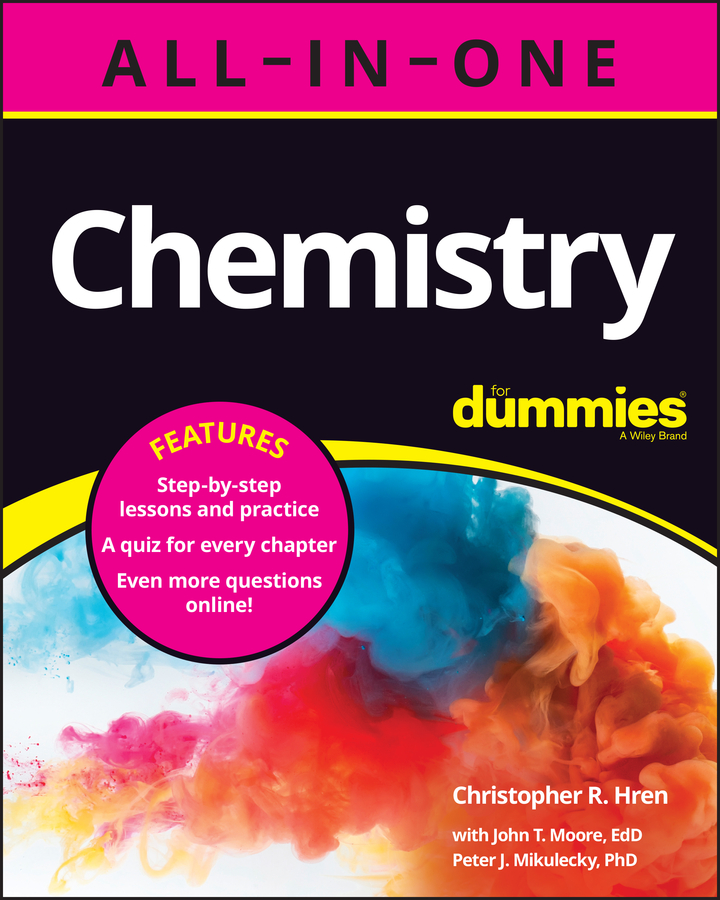Because spectator ions don't actually participate in the chemistry of a reaction, you don't always need to include them in a chemical equation. Doing so leads to a needlessly complicated reaction equation, so chemists often prefer to write net ionic equations, which omit the spectator ions. A net ionic equation doesn't include every component that may be present in a given beaker. Rather, it includes only those components that actually react.

Here's a simple recipe for making net ionic equations of your own:

1. Examine the starting equation to determine which ionic compounds are dissolved, as indicated by the (aq) symbol following the compound name.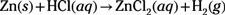2. Rewrite the equation, explicitly separating dissolved ionic compounds into their component ions.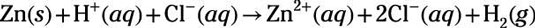Note that polyatomic ions don't break apart in solution, so be sure to familiarize yourself with the common ones.

3. Compare the reactant and product sides of the rewritten reaction and cross out the spectator ions.

Any dissolved ions that appear in the same form on both sides are spectator ions. Cross out the spectator ions to produce a net reaction. If all reactants and products cross out, then no reaction will occur.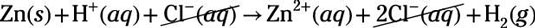The net reaction is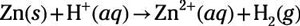As written, the preceding reaction is imbalanced with respect to the number of hydrogen atoms and the amount of positive charge.

4. Balance the net reaction for mass and charge.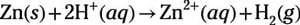If you want, you can balance the equation for mass and charge first (at Step 1). This way, when you cross out spectator ions at Step 3, you cross out equivalent numbers of ions. Either method produces the same net ionic equation in the end. Some people prefer to balance the starting reaction equation, but others prefer to balance the net reaction because it's a simpler equation.

Now apply these steps by generating a balanced net ionic equation for the following reaction: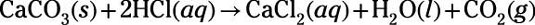Because HCl and CaCl2 are listed as aqueous (aq), rewrite the equation, explicitly separating those compounds into their ionic components: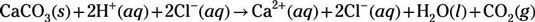Next, cross out any components that appear in the same form on both sides of the equation. In this case, the chloride ions (Cl) are crossed out: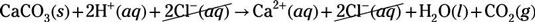This leaves the net reaction: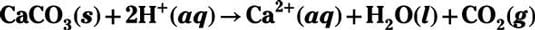The net reaction turns out to be balanced for mass and charge, so it's the balanced net ionic equation.